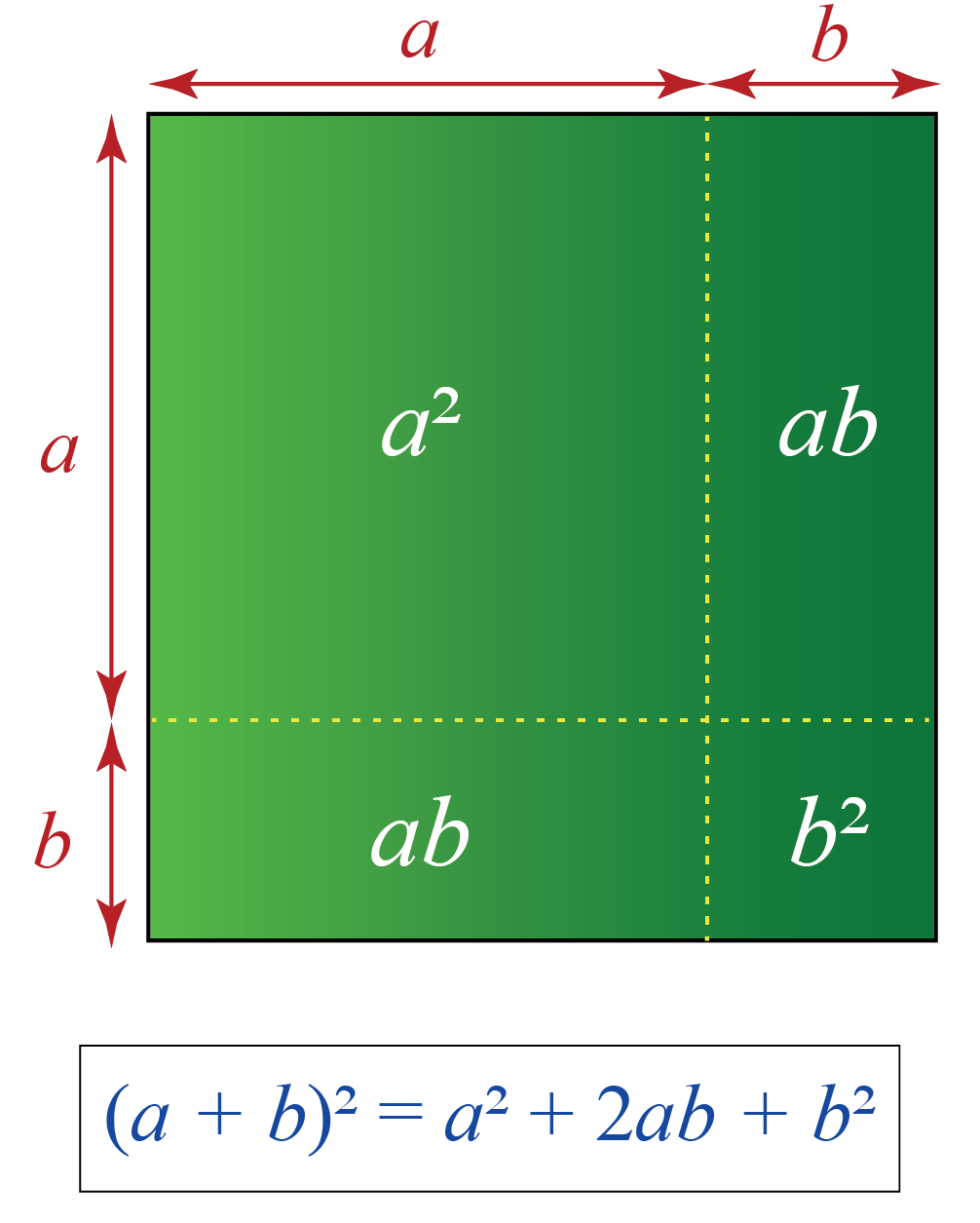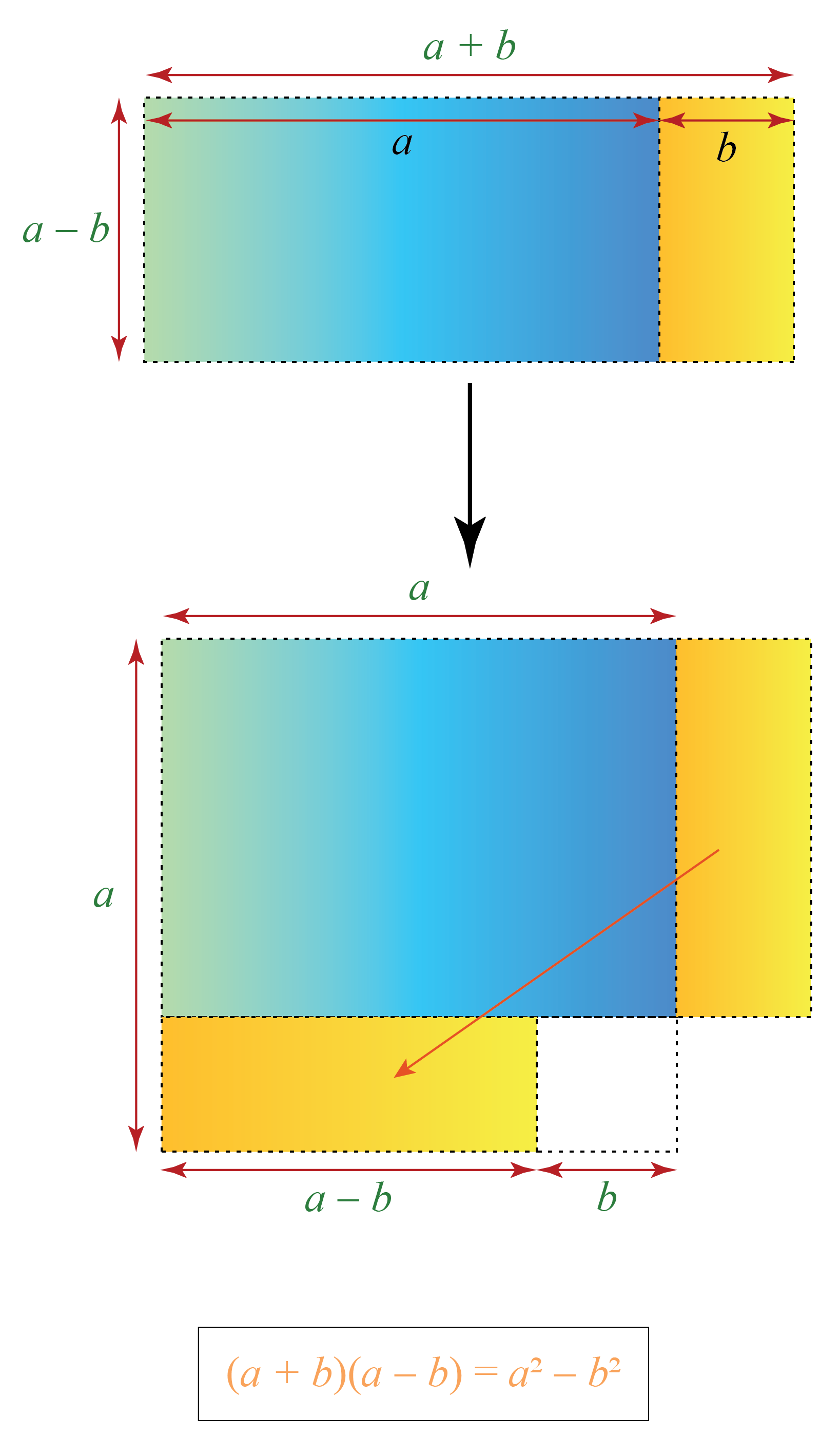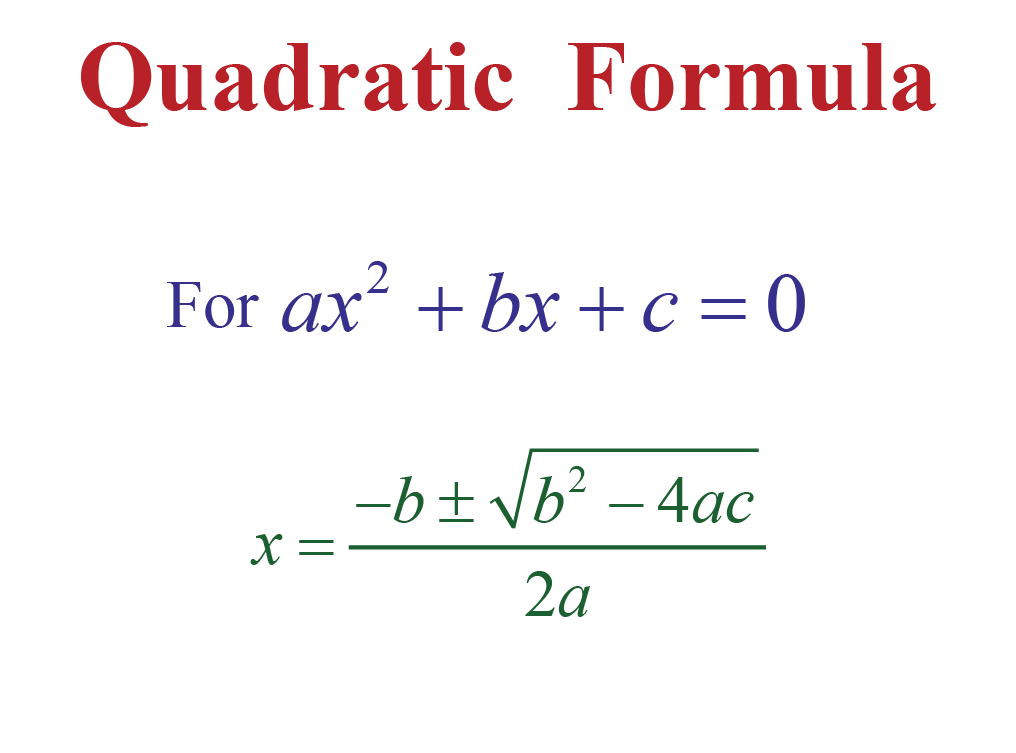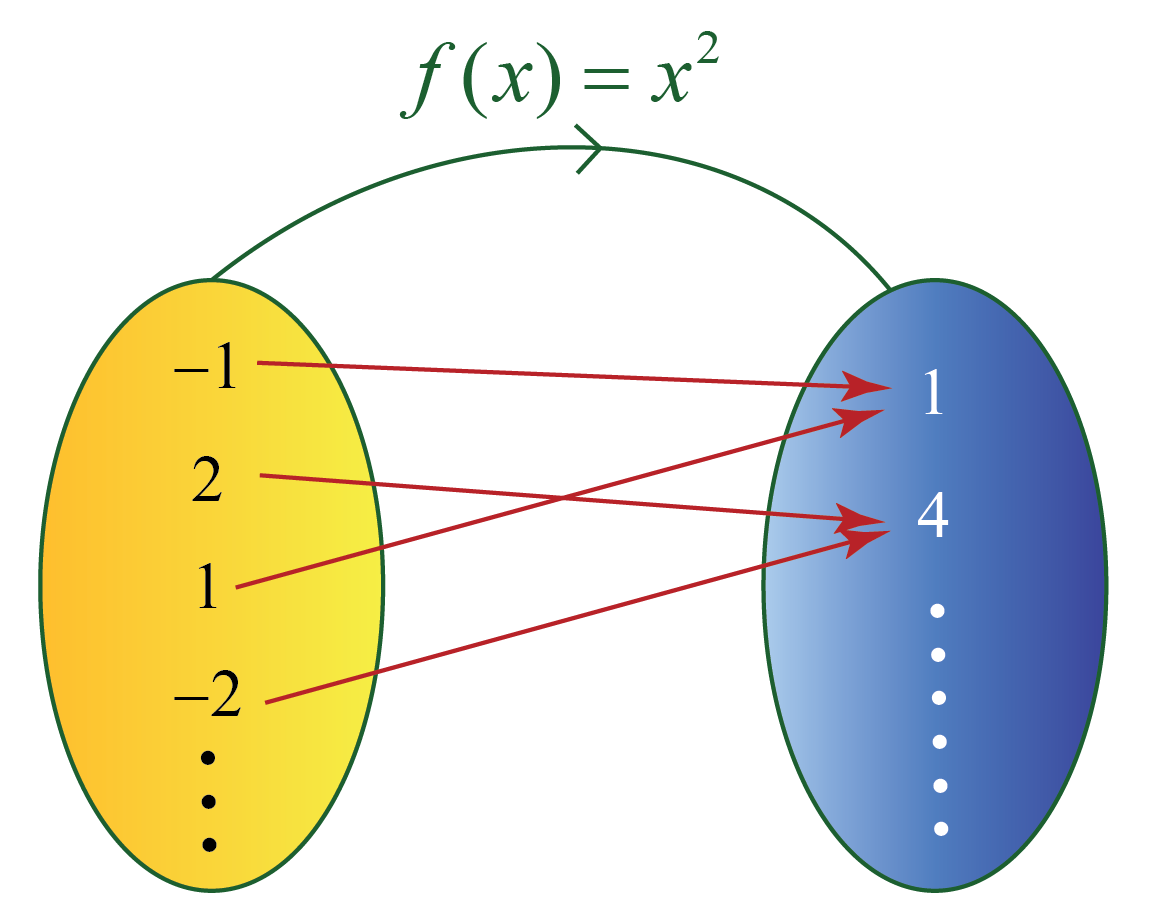# Algebraic Formulas

Go back to  'Algebra'

 1 Algebraic Identities 2 Tips and Tricks on Algebraic Identities 3 Algebraic Formulas 4 Algebra Formulas for Class 8 5 Algebra Formulas for Class 9 6 Algebra Formulas for Class 10 7 Algebra Formulas for Class 11 8 Algebra Formulas for Class 12 9 Algebraic Function 10 Algebraic Fractions 11 Solved Examples on Algebraic Formulas 12 Practice Questions on Algebraic Formulas 13 Challenging Questions on Algebraic Formulas 14 Maths Olympiad Sample Papers 15 Frequently Asked Questions (FAQs)

We at Cuemath believe that Math is a life skill. Our Math Experts focus on the “Why” behind the “What.” Students can explore from a huge range of interactive worksheets, visuals, simulations, practice tests, and more to understand a concept in depth.

Book a FREE trial class today! and experience Cuemath’s LIVE Online Class with your child.

## Algebraic Identities

• An identity is an equation that is always true regardless of the values assigned to the variables.
• Algebraic Identity means that the left-hand side of the equation is identical to the right-hand side, for all values of the variables.

Here are some most commonly used algebraic identities:

 Algebraic Identities $\begin{array}{l} (a+b)^{2} &=a^{2}+2 a b+b^{2} \\ (a-b)^{2} &=a^{2}-2 a b+b^{2} \\ (a+b)(a-b) &=a^{2}-b^{2} \\ (x+a)(x+b) &=x^{2}+x(a+b)+a b \end{array}$

### Proof of (a+b)2 = a2 + 2ab + b2

We can understand how the above formula is derived from the following figure:Still confusing?? Then we can use the following simulation to understand this in a more visual way.

### Proof of (a + b) (a - b) = a2 - b2

We can understand how the above formula is derived from the following figure:Still confusing? Then you can use the following simulation to understand this in a more visual way.

CLUEless in Math? Check out how CUEMATH Teachers will explain Algebraic Formulas to your kid using interactive simulations & worksheets so they never have to memorise anything in Math again!

Explore Cuemath Live, Interactive & Personalised Online Classes to make your kid a Math Expert. Book a FREE trial class today!Tips and Tricks
1. You can memorize the algebraic identities by understanding how they are derived.
For example,
\begin{align}(a+b)^2&=(a+b)(a+b)\\[0.2cm] &= a^2+ab+ab+b^2 \\[0.2cm] &= a^2+2ab+b^2\end{align}
In the same way, you can try to derive the other algebraic identities as well.

## Algebraic Formulas

An algebraic formula is an equation that is a rule written using mathematical and algebraic symbols.

It is an equation that involves algebraic expressions on both sides.

Example:

$$(a+b)^{2}=a^{2}+2 a b+b^{2}$$ is an algebraic formula and here,

## Algebra Formulas for Class 8

The algebraic formulas that are involved in class 8 are:

 Algebraic Formulas \begin{align} (a+b)^2 &= a^2+2ab+b^2\\[0.3cm] (a-b)^2 &=a^2-2ab+b^2 \\[0.3cm] a^2-b^2&= (a+b)(a-b)\\[0.3cm] (a+b)^3 &= a^3+3a^2b+3ab^2+b^3\\[0.3cm] (a-b)^3 &= a^3-3a^2b+3ab^2-b^3\\[0.3cm] a^3+b^3&= (a+b) (a^2-ab+b^2)\\[0.3cm] a^3-b^3&= (a-b) (a^2+ab+b^2)\\[0.3cm] (a+b+c)^2 &= a^2+b^2+c^2+2ab+2bc+2ca \end{align}

Some laws of exponents are:

 Laws of Exponents \begin{align} a^m \cdot a^n& = a^{m+n}\\[0.3cm] \frac{a^m}{a^n} &= a^{m-n}\\[0.3cm] (a^m)^n &= a^{mn}\\[0.3cm] (ab)^m &= a^m \cdot b^m \\[0.3cm] a^0 &=1\\[0.3cm] a^{-m} &= \frac{1}{a^m} \end{align}

## Algebra Formulas for Class 9

"Logarithms" is introduced in class 9 and here are some formulas related to this concept.

 Properties of Logarithms \begin{align} x^m =a &\Rightarrow \log_x a = m\\ \log _{a} 1 &=0\\ \log _{a} a &=1\\ \log _{a}(xy) &=\log _{a} x+\log _{a} y\\ \log _{a} \frac{x}{y} &=\log _{a} x-\log _{a} y\\ \log _{a}\left(x^{m}\right) &=m \log _{a} x\\ \log _{a} x &=\frac{\log _{c} x}{\log _{c} a}\\ a^{\log _{a} x} &=x \end{align}

## Algebra Formulas for Class 10

An important algebra formula in class 10 is the “quadratic formula”.

The “quadratic formula” is used to find the roots of a quadratic equation $$ax^2+bx+c=0$$.

This formula says:

 $x=\frac{-b \pm \sqrt{b^{2}-4 a c}}{2 a}$Here:

• If $$b^2-4ac>0$$ then the quadratic equation has two distinct real roots.
• If $$b^2-4ac=0$$ then the quadratic equation has two equal real roots.
• If $$b^2-4ac<0$$ then the quadratic equation has two imaginary roots.

Apart from this, we have a few other formulas related to progressions.

 1) nth term of an arithmetic sequence; $${a_n} = a+(n-1)d$$ 2) Sum of n terms of an arithmetic sequence; $$S_n = \frac{n}{2} (2a+(n-1)d)$$ 3) nth term of a geometric sequence; $$a_n = a \cdot r^{n-1}$$ 4) Sum of n terms of a geometric sequence; $$S_n = \frac{a\left(1-r^{n}\right)}{1-r}, r \neq 1$$ 5) Sum of infinite terms of a geometric sequence; $$S = \frac{a}{1-r}$$

## Algebra Formulas for Class 11

The algebraic formulas that are involved in class 11 are as follows:

 Algebraic Formulas \begin{align} n! &= n \times (n-1) \times …. 3 \times 2 \times 1\\[0.3cm] _{n} C_{r}&=\frac{n !}{(n-r) ! r !}\\[0.3cm] _{n} P_{r}&=\frac{n !}{(n-r) !} \end{align}

Apart from these, there is “Binomial Theorem” as well which is used to evaluate the large exponents of algebraic expressions with two terms. It says:

$$(x+y)^{n}=^{n} C_{0} x^{n} y^{0}+^{n} C_{1} x^{n-1} y^{1}+^{n} C_{2} x^{n-2} y^{2}+\dots+^{n} C_{n-1} x y^{n-1}+^{n} C_{n} x^{0} y^{n}$$

## Algebra Formulas for Class 12

The vector algebra formulas that are involved in class 12 are as follows.

For any three vectors, $$\vec{a}, \vec{b}$$ and $$\vec{c}$$:

• The magnitude of $$\vec{a} = x \hat{i}+y \hat{j}+z \hat{k}$$ is, $$|\vec{a}| = \sqrt{x^2+y^2+z^2}$$.

• The unit vector along $$\vec{a}$$ is $$\dfrac{\vec{a}}{|\vec{a}|}$$.

• The dot product is defined as: $$\vec{a} \cdot \vec{b} = |\vec{a}| |\vec{b}| \cos \theta$$, where $$\theta$$ is the angle between the vectors $$\vec{a}$$ and $$\vec{b}$$.

• The cross product is defined as: $$\vec{a} \times \vec{b} = |\vec{a}| |\vec{b}| \sin \theta \,\,\hat{n}$$, where $$\theta$$ is the angle between the vectors $$\vec{a}$$ and $$\vec{b}$$.

• The scalar triple product of three vectors is given by $$[\vec{a} \text{ } \vec{b} \text{ } \vec{c} ] = \vec{a} \cdot (\vec{b} \times \vec{c}) = (\vec{a} \times \vec{b}) \cdot \vec{c}$$.

## Algebraic Function

An algebraic function is of the form $$y=f(x)$$, where

• $$x$$ is called the input.
• $$y$$ is called the output.

Here, each input corresponds to exactly one output.

But multiple inputs may correspond to a single output.

For example:

$$f(x)= x^2$$ is an algebraic function.

Here, when $$x=2$$, $$f(2)= 2^2=4$$.

Here, $$x=2$$ is the input and $$f(2)=4$$ is the output of the function.## Algebraic Fractions

We can add, subtract, multiply, and divide fractions in algebra just the same way we do with fractions involving numbers.

$\frac{x}{y}+\frac{z}{w}=\frac{x w+y z}{y w}$

### Subtracting Fractions

$\frac{x}{y}-\frac{z}{w}=\frac{x w-y z}{y w}$

### Multiplying Fractions

$\frac{x}{y} \times \frac{z}{w}=\frac{x z}{y w}$

### Dividing Fractions

$\frac{x}{y} \div \frac{z}{w}=\frac{x w}{y z}$

## Solved Examples

Here are a few problems on algebra along with their solutions for you.

 Example 1

Using algebraic identities, find $$(2x-3y)^2$$.

Solution:

Here, we use the identity $$(a-b)^2= a^2-2ab+b^2$$ to expand this.

Here, $$a= 2x$$ and $$b=3y$$.

Then we get:

\begin{align} (2x-3y)^2 &= (2x)^2 -2(2x)(3y)+(3y)^2 \\ &= 4x^2 -12xy+9y^2 \end{align}

 $$\therefore (2x-3y)^2=4x^2 -12xy+9y^2$$
 Example 2

Using identities, evaluate $$297 \times 303$$.

Solution:

The above product can be written as $$(300-3) \times (300+3)$$.

We will find this product using the formula:

$(a-b)(a+b)=a^{2}-b^{2}$

Here $$a=300$$ and $$b=3$$.

Then we get:

\begin{aligned} (300-3) \times(300+3) &=300^2-3^2 \\ &=90000-9 \\ &=89991 \end{aligned}

 $$\therefore 297 \times 303= 89991$$
 Example 3

Find the roots of the quadratic equation $$x^2+5x+6=0$$ using the quadratic formula.

Solution:

The given equation is $$x^2+5x+6=0$$.

Comparing this with $$ax^2+bx+c=0$$, we get:

$a=1; \,\, b=2; \,\, c=2$.

Substituting these values in the quadratic formula:

\begin{align} x&=\frac{-b \pm \sqrt{b^{2}-4 a c}}{2 a}\\[0.2cm] x&=\frac{-5 \pm \sqrt{5^2-4 \cdot 1 \cdot 6}}{2 \cdot 1}\\[0.2cm] x&=\frac{-5 \pm \sqrt{1}}{2 \cdot 1}\\[0.2cm] x&=\frac{-5 \pm 1}{2}\\[0.2cm] x &= \frac{-5+1}{2}, \,\,\, x=\frac{-5-1}{2}\\[0.2cm] x &= -2; \,\,\, x=-3 \end{align}

 $$\therefore x= -2, -3$$
 Example 4

Use the laws of exponents and simply the following expression:

$\left(\frac{6 \times 10}{2^{2} \times 5^{3}}\right)^{2} \times \frac{25}{27}$

Solution:

We will simplify the given expression using the laws of exponents:

 \begin{aligned} \left(\frac{6 \times 10}{2^{2} \times 5^{3}}\right)^{2} \times \frac{25}{27} &=\left(\frac{2 \times 3 \times 2 \times 5}{2^{2} \times 5^{3}}\right)^{2} \times \frac{5^{2}}{3^{3}} \quad[\because 6=2 \times 3 \text { and } 10=2 \times 5] \0.3cm] &=\left(\frac{2^{2} \times 3 \times 5}{2^{2} \times 5^{3}}\right)^{2} \times \frac{5^{2}}{3^{3}} \\[0.3cm]\ &=\left(\frac{3}{5^{2}}\right)^{2} \times \frac{5^{2}}{3^{3}} \left [\because(a \times b)^{m}=a^{m} \times b^{m}\right] \\[0.3cm] &=\frac{3^{2}}{5^{4}} \times \frac{5^{2}}{3^{3}} \\[0.3cm] &=\frac{1}{5^{2} \times 3} \\[0.3cm] &=\frac{1}{25 \times 3} \\[0.3cm] &=\frac{1}{75} \quad\left[\because\left(a^{m}\right)^{n}=a^{m n}\right] \end{aligned}  $$\therefore \left(\frac{6 \times 10}{2^{2} \times 5^{3}}\right)^{2} \times \frac{25}{27} = \dfrac{1}{75}$$  Example 5 Compress the following expression into a single logarithm using the properties of logarithms: \[ 5 \log _{3}(x)+2 \log _{3}(A x)-\log _{3}\left(8 x^{5}\right)

Solution:

We will compress the given expression using the properties of logarithms:

 \begin{align}5 \log _{3}(x)+2 \log _{3}(4 x)-\log _{3}\left(8 x^{5}\right) &=\log _{3}\left(x^{5}\right)+\log _{3}\left( (4 x)^{2}\right)-\log _{3}\left(8 x^{5}\right) \0.3cm] &=\log _{3}\left(x^{5}\right)+\log _{3}\left(16 x^{2}\right)-\log _{3}\left(8 x^{5}\right) \\[0.3cm] &=\log _{3}\left(x^{5} \cdot 16 x^{2}\right)-\log _{3}\left(8 x^{5}\right) \\[0.3cm] &=\log _{3}\left(16 x^{7}\right)-\log _{3}\left(8 x^{5}\right) \\[0.3cm] &=\log _{3}\left(\frac{16 x^{7}}{8 x^{5}}\right)\\[0.3cm] &= \log _{3}\left(2 x^{2}\right) \end{align}  $$\therefore 5 \log _{3}(x)+2 \log _{3}(A x)-\log _{3}\left(8 x^{5}\right) = \log _{3}\left(2 x^{2}\right)$$ Help your child score higher with Cuemath’s proprietary FREE Diagnostic Test. Get access to detailed reports, customised learning plans and a FREE counselling session. Attempt the test now. ## Practice Questions Here are a few problems related to the algebraic formulas. Select/Type your answer and click the "Check Answer" button to see the result.Challenging Questions 1. Find the roots of the quadratic equation: \[x^2+7x+12=0
2. Simplify the expression:

$\frac{x^{-9} y^{3}}{x^{-7} y^{8}}$

so that the answer has no negative exponents.

3. Expand the logarithm:

$\log (x^2 y^3 z)$

IMO (International Maths Olympiad) is a competitive exam in Mathematics conducted annually for school students. It encourages children to develop their math solving skills from a competition perspective.

## 1.  What are the formulas in algebra?

Here are some most commonly used formulas of algebra:

• $$\begin{equation}a^{2}-b^{2}=(a-b)(a+b)\end{equation}$$
• $$\begin{equation}(a+b)^{2}=a^{2}+2 a b+b^{2}\end{equation}$$
• $$\begin{equation}(a-b)^{2}=a^{2}-2 a b+b^{2}\end{equation}$$
• $$(x+a)(x+b)=x^{2}+x(a+b)+a b$$
• $$\begin{equation}(a+b+c)^{2}=a^{2}+b^{2}+c^{2}+2 a b+2 b c+2 c a\end{equation}$$
• $$\begin{equation}(a+b)^{3}=a^{3}+3 a^{2} b+3 a b^{2}+b^{3}\end{equation}$$
• $$\begin{equation}(a-b)^{3}=a^{3}-3 a^{2} b+3 a b^{2}-b^{3}\end{equation}$$
• Laws of Exponents:\begin{align} a^m \cdot a^n& = a^{m+n}\\[0.3cm] \frac{a^m}{a^n} &= a^{m-n}\\[0.3cm] (a^m)^n &= a^{mn}\\[0.3cm] (ab)^m &= a^m \cdot b^m \\[0.3cm] a^0 &=1\\[0.3cm] a^{-m} &= \frac{1}{a^m} \end{align}

• The quadratic formula says the roots of the equation $$a x^{2}+b x+c=0$$ where $$\begin{equation}a \neq 0\end{equation}$$ are given by:

$x=\frac{-b \pm \sqrt{b^{2}-4 a c}}{2 a}$

## 2. What are the basic math formulas?

Here are some basic math formulas:

• $$\begin{equation}a^{2}-b^{2}=(a-b)(a+b)\end{equation}$$
• $$\begin{equation}(a+b)^{2}=a^{2}+2 a b+b^{2}\end{equation}$$
• $$\begin{equation}(a-b)^{2}=a^{2}-2 a b+b^{2}\end{equation}$$
• $$(x+a)(x+b)=x^{2}+x(a+b)+a b$$
• $$\begin{equation}(a+b+c)^{2}=a^{2}+b^{2}+c^{2}+2 a b+2 b c+2 c a\end{equation}$$
• $$\begin{equation}(a+b)^{3}=a^{3}+3 a^{2} b+3 a b^{2}+b^{3}\end{equation}$$
• $$\begin{equation}(a-b)^{3}=a^{3}-3 a^{2} b+3 a b^{2}-b^{3}\end{equation}$$

## 3. How do I learn algebraic formulas?

• Algebraic formulas can be easily memorized by visualising the formulas as squares or rectangles.
• It also helps by remembering the factored forms rather than the simplified form.

## 4. What is the formula for $$\begin{equation}a^{2}-b^{2}\end{equation}$$?

The formula for $$\begin{equation}a^{2}-b^{2}\end{equation}$$ is
$\begin{equation}(a+b)(a-b)=a^{2}-b^{2}\end{equation}$
It is called the difference of squares formula.

More Important Topics
Numbers
Algebra
Geometry
Measurement
Money
Data
Trigonometry
Calculus